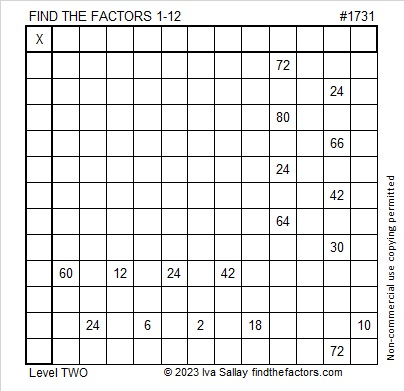# 1731: The Sum of the Squares of Three Consecutive Prime Numbers

Contents

### Today’s Puzzle:

Write the numbers from 1 to 12 in both the first column and the top row so that the given clues are the products of the numbers you write.### Factors of 1731:

• 1731 is a composite number.
• Prime factorization: 1731 = 3 × 577.
• 1731 has no exponents greater than 1 in its prime factorization, so √1731 cannot be simplified.
• The exponents in the prime factorization are 1 and 1. Adding one to each exponent and multiplying we get (1 + 1)(1 + 1) = 2 × 2 = 4. Therefore 1731 has exactly 4 factors.
• The factors of 1731 are outlined with their factor pair partners in the graphic below.### More About the Number 1731:

1731 is the hypotenuse of a Pythagorean triple:
144-1725-1731 which is 3 times (48-575-577).

OEIS.org informs us that 1731 is the sum of the squares of three consecutive prime numbers. Let’s find those three prime numbers. Since √(1731/3) rounds to 24, I’m guessing the middle prime number is 23. The prime numbers occurring before and after it are 19 and 29.

Is 19² + 23² + 29² = 1731? Yes, it is!

Here are some other ways that 1731 is the sum of three squares:
41² + 7² + 1² = 1731,
41² + 5² + 5² = 1731,
37² + 19² + 1² = 1731, and
29² + 29² + 7² = 1731.

This site uses Akismet to reduce spam. Learn how your comment data is processed.